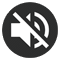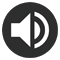# 10 Times table Chart - Printable PDF

3 Reviews

## Print the 10 Times table Chart

A pretty 10 Times table chart in A4 format (PDF). You can see that the 10 times table is really easy, so you don't need to learn it by heart!

Print for free this 10 times table and don't forget to take a look at the time-saving trick for the 10 times table. Then, practice online the 10 times table.

This chart is also available in other colors or in black and white. Feel free to look at all the templates we propose below and choose your favorite one!
In a Multiplication Chart, the results are displayed in a table (with columns and rows), whereas in a Times table Chart they are displayed as a list of increasing multiplications.

In the 10 times table, you will find the following multiplications:

10x1=10, 10x2=20, 10x3=30, 10x4=40, 10x5=50, 10x6=60, 10x7=70, 10x8=80, 10x9=90, 10x10=100, 10x11=110, 1x12=120

Print the 10 Times table Chart (PDF)

If you plan to print the other times tables, we advise you to choose a different color for each multiplication table from 1 to 12. To save time, you will find, in our All Times tables section, PDFs including all the tables colored like a rainbow.

## Our other 10 Times table PDF

Thanks to the many colors available, you will undoubtedly find your favorite chart! Our black and white 10 Times table charts will be perfect if you prefer sobriety, if you want to save ink or if you prefer to color your times table yourself using your pencils or markers. Feel free to click on the images to preview the PDF or use the "PRINT" button below the image to download the chart of your choice.

10 Times table Chart
Black & white
Print
10 Times table Chart
Handwritten numbers
Print
10 Times table Chart
Ink saving
Print
10 Times table Chart
Grey
Print
10 Times table Chart
Dark green
Print
10 Times table Chart
Green
Print
10 Times table Chart
Light green
Print
10 Times table Chart
Turquoise
Print
10 Times table Chart
Blue
Print
10 Times table Chart
Dark blue
Print
10 Times table Chart
Purple
Print
10 Times table Chart
Pink
Print
10 Times table Chart
Light pink
Print

## Trick for the 10 times table

The 10 times table is really very simple! There's no multiplication to memorize! You just need to understand a very simple rule: when you multiply a number by 10, just add a zero after the number to obtain the result of the multiplication. To help you understand, take a look at the examples below.

### The results of the 10 times table

• 10 x 1 = 10
• 10 x 2 = 20
• 10 x 3 = 30
• 10 x 4 = 40
• 10 x 5 = 50
• 10 x 6 = 60
• 10 x 7 = 70
• 10 x 8 = 80
• 10 x 9 = 90
• 10 x 10 = 100

### Mutiply by 10

The above rule applies in the same way whatever number is multiplied by 10. Here are a few examples:

• 10 x 97 = 970
• 10 x 200 = 2000
• 10 x 845 = 8450
• 10 x 1399 = 13990
• 10 x 10000 = 100000
• ...

### Mutiply by 100

If you multiply a number by 100, it's the same thing except that you add 2 zeros at the end. If you multiply by 1000, you add 3 zeros and so on... Here are a few examples:

• 100 x 8 = 800
• 100 x 50 = 5000
• 100 x 125 = 12500
• 100 x 999 = 99900
• 100 x 1400 = 140000
• ...
/   Publié par Memozor
Dernière modification le 20 septembre 2023

## Online exercise for the 10 times table

In this exercise, you can choose between several settings, so it's up to you to select the ones that suit you best. Here are the available options:

• Number color: By choosing the colorful numbers option, the color code of the table of 10 (light pink) is applied. This is the same color code as the printable 10 times table chart at the top of the page.
• Number format: you can choose a specific font for the numbers. There are standard fonts, but also school fonts. Select the one that's closest to the one used at school.
• Game mode: random or increasing.

If you like this exercise, you can also practice with the other tables in the All times tables section.

USEFUL !
You can see your more common mistakes below the settings.NUMBERS :
NUMBER FORMAT :
MULTIPLICATIONS :
GAME MODE :

### Your most common mistakes :

You will find here the list of your most common mistakes

### A chart with all the times tables (2 PDF to cut and assemble)

A pretty chart with all the times tables to print in A4 format (PDF). We have assigned a different color code for each times table, with a nice color gradient. The colors will help you memorize all your multiplication tables from the 1 to the 12 times table (other colors are available for this resource).

See the Chart